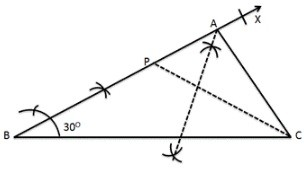# Chapter 11 Constructions RS Aggarwal Solution for Class 9th Maths

0
1255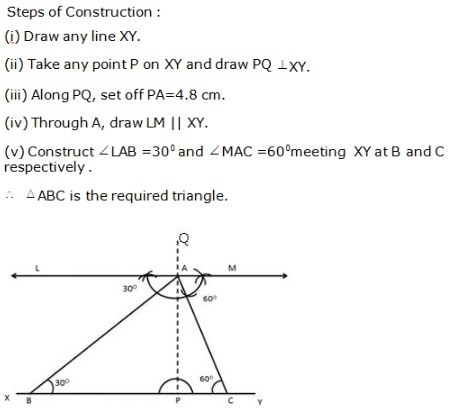GeometricalConstructions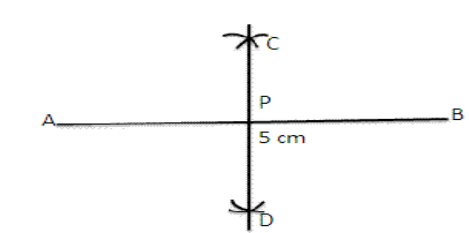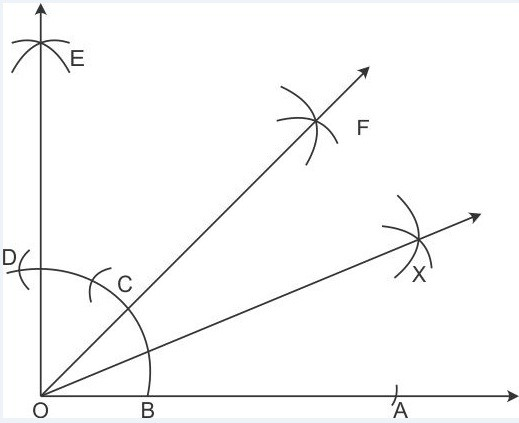Exercise 12A

# Question 1:

Steps of Construction:

1. Draw a line segment AB = 5 cm
2. With A as centre and radius equal to more than half of AB, draw two arcs, one above AB and the other below AB.
3. With B as a centre and the same radius draw two arcs which cuts the previously drawn arcs at C and D.
4. Join CD , intersecting AB at point P.

∴ CD is the perpendicular bisector of AB at the point P.

# Question 2:

Step of Construction:

1. Draw a line segment OA.
2. AT A, draw ∠AOE=90 , using ruler and compass.
3. With B as centre and radius more than half of BD, draw an arc.
4. With D as centre and same radius draw another arc which cuts the previous arc at F.
5. Join OF. ∴ ∠AOF=45 .
6. Now with centre B and radius more than half of BC, draw an arc.
7. With centre C and same radius draw another arc which cuts the previously drawn arc at X.
8. Join OX. ∴ OX is the bisector of ∠AOF.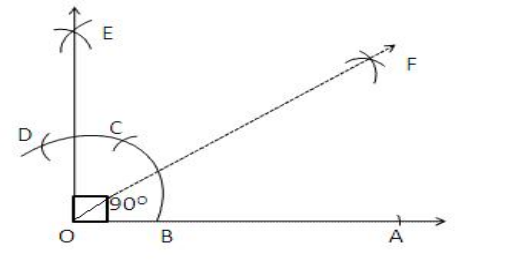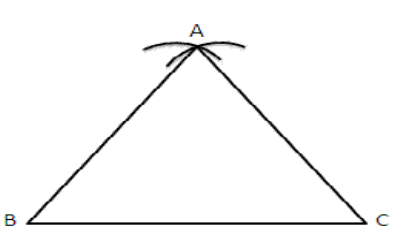# Question 3:

Step of Construction:

1. Draw a line segment OA.
2. With O as centre and any suitable radius draw an arc, cutting OA at B.
3. With B as centre and the same radius cut the previously drawn arc at C.
4. With C as centre and the same radius cut the arc at D.
5. With C as centre and the radius more than half CD draw an arc.
6. With D as centre and the same radius draw another arc which cuts the previous arc at E.
7. Join E Now, ∠AOE =900
8. Now with B as centre and radius more than half of CB draw an arc.

(iv) With C as centre and same radius draw an arc which cuts the previous at F.

1. Join OF.
2. ∴ F is the bisector of right ∠AOE.

# Question 4:

Step of construction:

1. Draw a line segment BC=5cm.
2. With B as centre and radius equal to BC draw an arc.
3. With C as centre and the same radius draw another arc which cuts the previous arc at A.
4. Join AB and AC.

Then ∆ABC is the required equilateral triangle.

#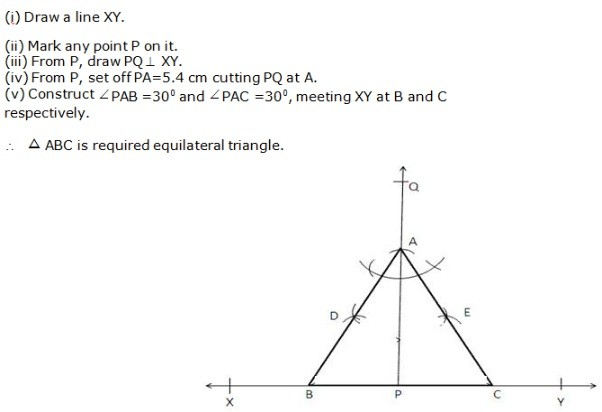Question 5: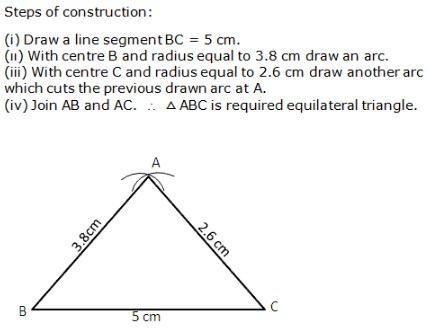Question 6:

Question 7: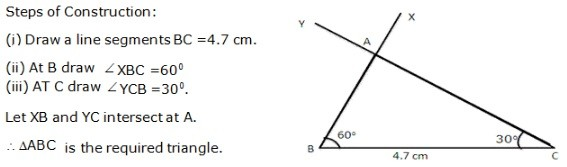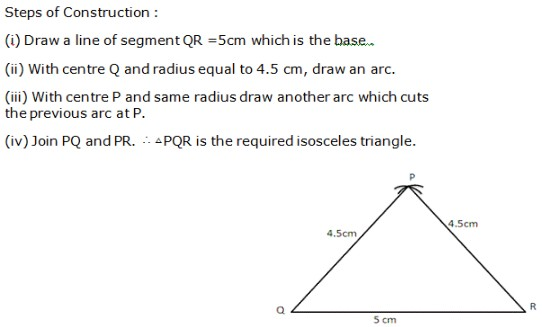Question 8: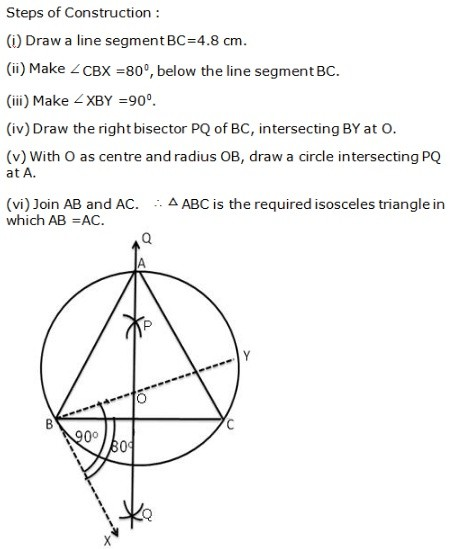Question 9:

Question 10: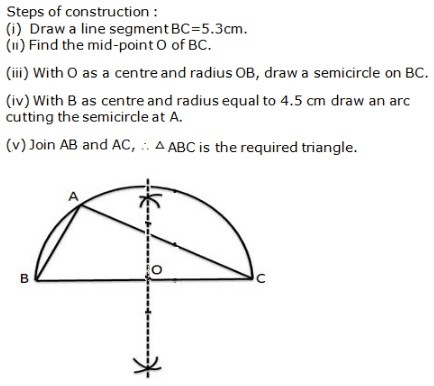Question 11:

Question 12: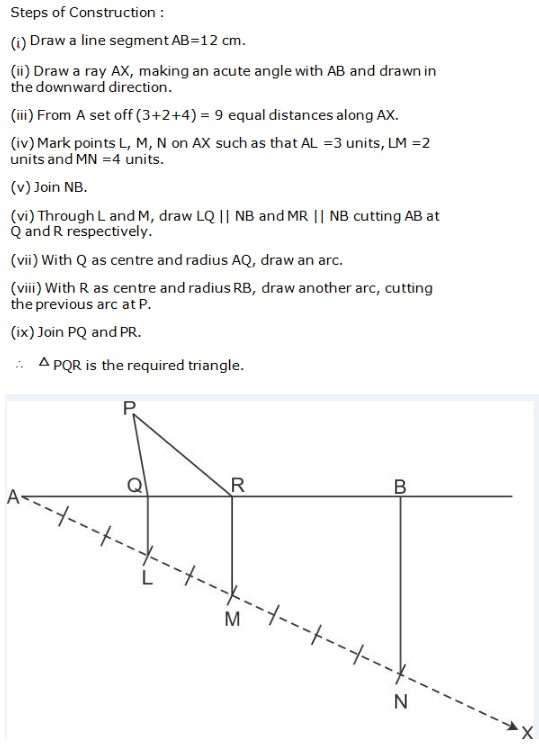Question 13:

Steps of Construction:

1. Draw BC = 4.5 cm.
2. Construct ∠CBX = 600
3. Along BX set off BP =8cm.
4. Join CP.
5. Draw the perpendicular bisector of CP to intersecting BP at A.
6. Join AC. ∴ ∆ABC is the required triangle.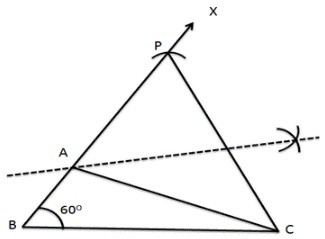# Question 14:

Steps of Construction:

1. Draw BC = 5.2 cm.
2. Construct ∠CBX = 300
3. Set off BP = 3.5 cm.
4. Join PC.
5. Draw the right bisector of PC, meeting BP produced at A.
6. Join AC. ∴ ∆ABC is the required triangle.